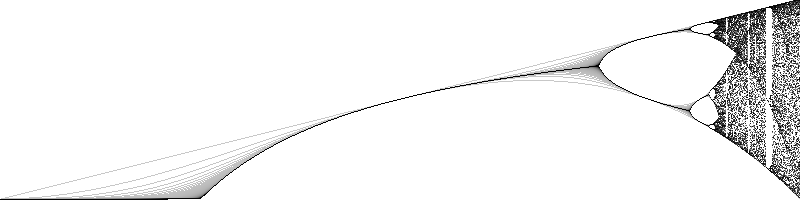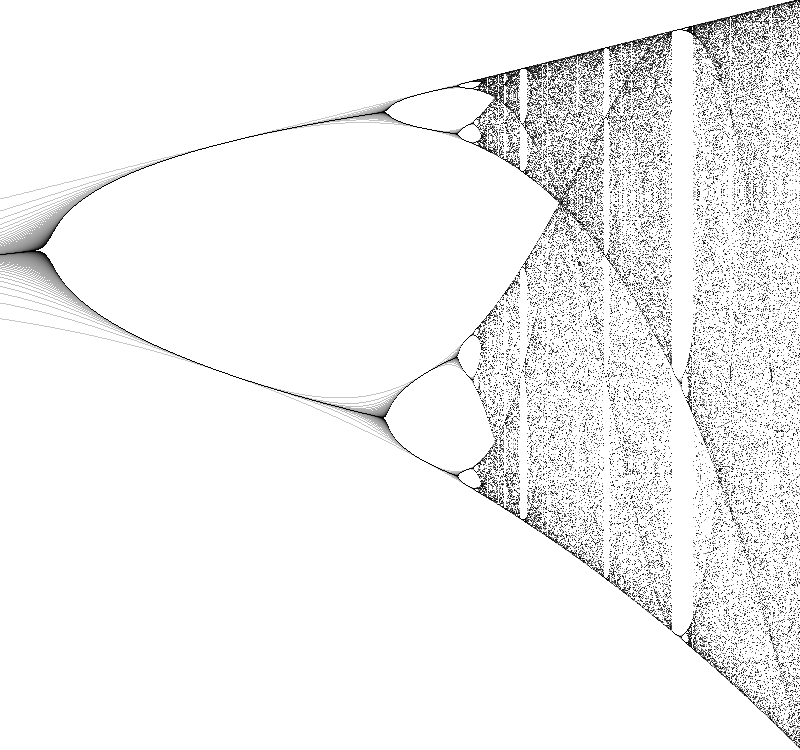# Logistic map

### Rendered in Python, explained in English

##### By Dany Shaanan
The Logistic map is an example of how chaotic behaviour can emerge from simple circumstances.

For values of r between 0 and 4 (left to right), we iterate the following function: (starting with x = 0.5)

x <- r * x * (1-x)

For low values of r (left), x eventually sets to zero. For medium valus of r (middle), x eventually sets to some single value greater than zero. For higher values of r (right), x jumps between two values, then four, then eight, and eventually, goes crazy in a chaotic pattern.See the code here